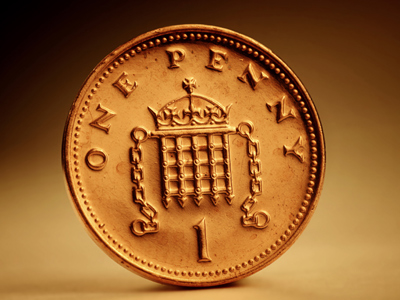There are 100 one penny pieces in a pound.

# Decimal Numbers (Year 4)

In KS2 Maths children will come to understand decimal numbers. In Year Four children will learn the initial place value of figures in decimal numbers - tenths. They will also do some basic adding and subtracting with decimals and be introduced to rounding decimal numbers up or down.

Decimal numbers are numbers that are not whole. 3, 7, 9 and 10 are whole numbers. 3.4, 7.1, 9.2 and 10.3 are decimal numbers. Can you spot the difference? The decimal numbers have a 'dot' which is called a decimal point. Adding and subtracting decimal numbers is just like doing it with whole numbers, for example 11 + 12 = 23 and 1.1 + 1.2 = 2.3. Decimal numbers can also be changed into the whole number they are closest to. We call this rounding up or down. For example, 4.7 would be rounded up to 5 but 4.4 would be rounded down to 4.

Play this quiz and find out how good you are at adding, subtracting and rounding decimal numbers.

1.
Which is the smallest?
1.7
0.9
2.1
0.3
310 is less than 910
2.
What is 345p in £s?
£3.45
£345
£0.345
£34.5
There are 100 pence in £1 so we divide 345 by 100 which gives 3.45 or £3 and 45p
3.
What is £19.42 rounded to the nearest £?
£18
£19
£19.50
£20
We round down numbers less than .5 and we round up numbers equal to or higher than .5
4.
How is 0.8 changed to 0.2?
Subtract 0.6
Subtract 6
Just like 8 - 6 = 2
0.8 - 0.6 = 0.2
5.
Which is the decimal fraction equivalent to thirty two and five tenths?
3.25
32.5
0.325
325
Whole numbers are on the left of the decimal point
6.
How is 4.3 changed to 4.7?
Subtract 0.4
Divide by 0.4
Just like 43 + 4 = 47,
4.3 + 0.4 = 4.7
7.
What is the figure 7 worth in 1.7?
7 ones
7 hundredths
7 tenths
7 tens
A number 7 in the tenths column means 710
8.
What does the digit 4 represent in 3.4?
4 tenths
4 tens
4 ones
4 hundredths
Tenths are the column after the decimal point
9.
What is 423cm in metres?
423m
42.3m
4.23m
0.423m
There are 100cm in a metre. Therefore 423cm is 4m and 23cm or 4.23m
10.
Which is the largest?
2.9
3.4
4.3
0.8
All the other numbers have less than 4 ones